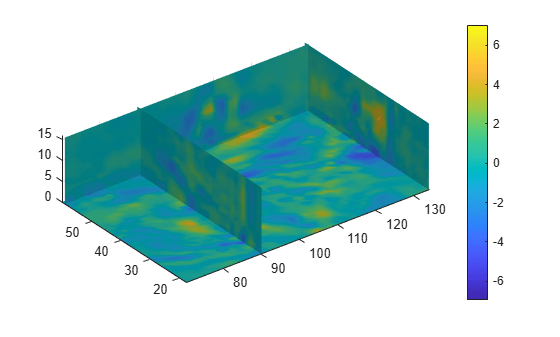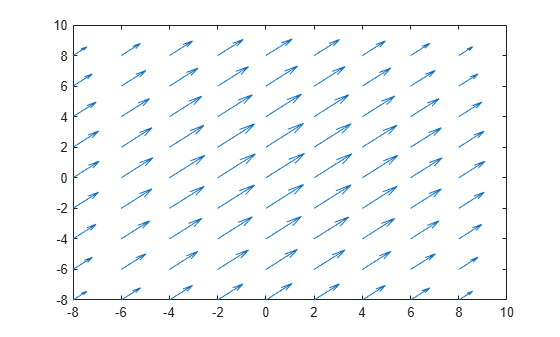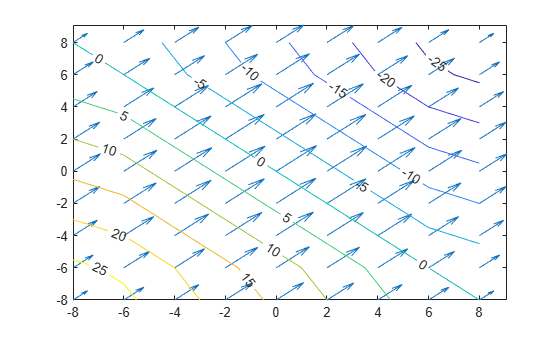# divergence

Compute divergence of vector field

## Syntax

``div = divergence(X,Y,Z,Fx,Fy,Fz)``
``div = divergence(Fx,Fy,Fz)``
``div = divergence(X,Y,Fx,Fy)``
``div = divergence(Fx,Fy)``

## Description

example

````div = divergence(X,Y,Z,Fx,Fy,Fz)` computes the numerical divergence of a 3-D vector field with vector components `Fx`, `Fy`, and `Fz`. The arrays `X`, `Y`, and `Z`, which define the coordinates for the vector components `Fx`, `Fy`, and `Fz`, must be monotonic, but do not need to be uniformly spaced. `X`, `Y`, and `Z` must be 3-D arrays of the same size, which can be produced by `meshgrid`.```
````div = divergence(Fx,Fy,Fz)` assumes a default grid of sample points. The default grid points `X`, `Y`, and `Z` are determined by the expression `[X,Y,Z] = meshgrid(1:n,1:m,1:p)`, where ```[m,n,p] = size(Fx)```. Use this syntax when you want to conserve memory and are not concerned about the absolute distances between points.```

example

````div = divergence(X,Y,Fx,Fy)` computes the numerical divergence of a 2-D vector field with vector components `Fx` and `Fy`. The matrices `X` and `Y`, which define the coordinates for `Fx` and `Fy`, must be monotonic, but do not need to be uniformly spaced. `X` and `Y` must be 2-D matrices of the same size, which can be produced by `meshgrid`.```
````div = divergence(Fx,Fy)` assumes a default grid of sample points. The default grid points `X` and `Y` are determined by the expression ```[X,Y] = meshgrid(1:n,1:m)```, where `[m,n] = size(Fx)`. Use this syntax when you want to conserve memory and are not concerned about the absolute distances between points.```

## Examples

collapse all

Load a 3-D vector field data set that represents a wind flow. The data set contains arrays of size 35-by-41-by-15.

`load wind`

Compute the numerical divergence of the vector field.

`div = divergence(x,y,z,u,v,w);`

Display the divergence of vector volume data as slice planes. Show the divergence at the $yz$-planes with $x=90$ and $x=134$, at the $xz$-plane with $y=59$, and at the $xy$-plane with $z=0$. Use color to indicate divergence.

```h = slice(x,y,z,div,[90 134],59,0); shading interp colorbar daspect([1 1 1]); axis tight camlight set([h(1),h(2)],'ambientstrength',0.6);```Specify 2-D coordinates and a vector field.

```[x,y] = meshgrid(-8:2:8,-8:2:8); Fx = 200 - (x.^2 + y.^2); Fy = 200 - (x.^2 + y.^2);```

Plot the vector field components `Fx` and `Fy`.

`quiver(x,y,Fx,Fy)`Find the numerical divergence of the 2-D vector field. Plot the contour of the divergence.

```D = divergence(x,y,Fx,Fy); hold on contour(x,y,D,'ShowText','on')```## Input Arguments

collapse all

Input coordinates, specified as matrices or 3-D arrays.

• For 2-D vector fields, `X` and `Y` must be 2-D matrices of the same size, and that size can be no smaller than `2`-by-`2`.

• For 3-D vector fields, `X`, `Y`, and `Z` must be 3-D arrays of the same size, and that size can be no smaller than `2`-by-`2`-by-`2`.

Data Types: `single` | `double`
Complex Number Support: Yes

Vector field components at the input coordinates, specified as matrices or 3-D arrays. `Fx`, `Fy`, and `Fz` must be the same size as `X`, `Y`, and `Z`.

Data Types: `single` | `double`
Complex Number Support: Yes

collapse all

### Numerical Divergence

The numerical divergence of a vector field is a way to estimate the values of the divergence using the known values of the vector field at certain points.

For a 3-D vector field of three variables $F\left(x,y,z\right)={F}_{x}\left(x,y,z\right)\text{\hspace{0.17em}}{\stackrel{^}{e}}_{x}+{F}_{y}\left(x,y,z\right)\text{\hspace{0.17em}}{\stackrel{^}{e}}_{y}+{F}_{z}\left(x,y,z\right)\text{\hspace{0.17em}}{\stackrel{^}{e}}_{z}$, the definition of the divergence of F is

For a 2-D vector field of two variables $F\left(x,y\right)={F}_{x}\left(x,y\right)\text{\hspace{0.17em}}{\stackrel{^}{e}}_{x}+{F}_{y}\left(x,y\right)\text{\hspace{0.17em}}{\stackrel{^}{e}}_{y}$, the divergence is

## Algorithms

`divergence` computes the partial derivatives in its definition by using finite differences. For interior data points, the partial derivatives are calculated using central difference. For data points along the edges, the partial derivatives are calculated using single-sided (forward) difference.

For example, consider a 2-D vector field F that is represented by the matrices `Fx` and `Fy` at locations `X` and `Y` with size `m`-by-`n`. The locations are 2-D grids created by `[X,Y] = meshgrid(x,y)`, where `x` is a vector of length `n` and `y` is a vector of length `m`. `divergence` then computes the partial derivatives Fx / ∂x and Fy / ∂y as

• `dFx(:,i) = (Fx(:,i+1) - Fx(:,i-1))/(x(i+1) - x(i-1))` and

`dFy(j,:) = (Fy(j+1,:) - Fy(j-1,:))/(y(j+1) - y(j-1))`

for interior data points.

• `dFx(:,1) = (Fx(:,2) - Fx(:,1))/(x(2) - x(1))` and

`dFx(:,n) = (Fx(:,n) - Fx(:,n-1))/(x(n) - x(n-1))`

for data points at the left and right edges.

• `dFy(1,:) = (Fy(2,:) - Fy(1,:))/(y(2) - y(1))` and

`dFy(m,:) = (Fy(m,:) - Fy(m-1,:))/(y(m) - y(m-1))`

for data points at the top and bottom edges.

The numerical divergence of the vector field is equal to ```div = dFx + dFy```.